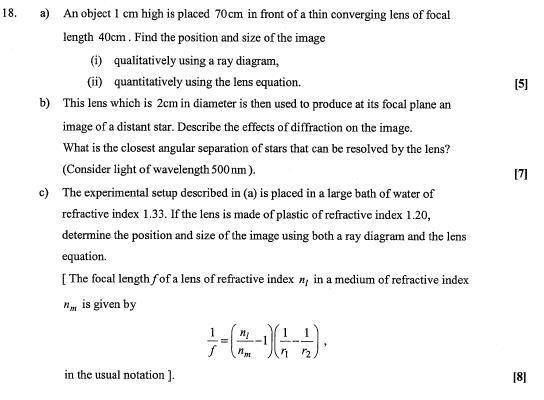# Lens question

## Homework Statement

the question is attached## The Attempt at a Solution

ok so a and b i can do but c), i donts know whether my answer is right and im a little confused about the conceptual idea...

so first thing i did was found the radius of curvature using the lens maker equ using the original setup in air...this i found to be 16cm... [note r1 = -r2]

next i found the focal length in the water situation (i know it doesn't ask for it but still)...this i found to be -81.8cm...
this confuses me cause i thought that only concave lens had negative focal lengths and this is a concave lens...also can the focal length be greater than the radius in a lens? i think it can but cant find anywhere that confirms this...

now to find the image distance s' ...... 1/f = 1/s + 1/s'

i got s' = -37.73cm

then magnification i can do but is what ive done ok?
also can you have a diverging lens that is concave if the object is infront of the focal length? cause that is the only reason that i can think for the focal length to be negative...

Last edited: